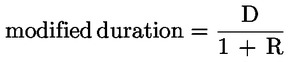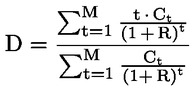CRR Tool
Capital Requirements Regulation (CRR)
Article 340

# Article 340 - Duration-based calculation of general risk

Status
In force
Selected consolidated version from
30/09/2021
Search all articles and keywords within this legal act

Article 340

Duration-based calculation of general risk

1.
Institutions may use an approach for calculating the own funds requirement for the general risk on debt instruments which reflects duration, instead of the approach set out in Article 339, provided that the institution does so on a consistent basis.
2.
Under the duration-based approach referred to in paragraph 1, the institution shall take the market value of each fixed-rate debt instrument and hence calculate its yield to maturity, which is implied discount rate for that instrument. In the case of floating-rate instruments, the institution shall take the market value of each instrument and hence calculate its yield on the assumption that the principal is due when the interest rate can next be changed.
3.

The institution shall then calculate the modified duration of each debt instrument on the basis of the following formula:where:

D

=

duration calculated according to the following formula:where:

 R = yield to maturity;
 Ct = cash payment in time t;
 M = total maturity.

Correction shall be made to the calculation of the modified duration for debt instruments which are subject to prepayment risk. EBA shall, in accordance with Article 16 of Regulation (EU) No 1093/2010, issue guidelines about how to apply such corrections.

4.

The institution shall then allocate each debt instrument to the appropriate zone in Table 3. It shall do so on the basis of the modified duration of each instrument.

Table 3

 Zone Modified duration (in years) Assumed interest (change in %) One > 0 ≤ 1,0 1,0 Two > 1,0 ≤ 3,6 0,85 Three > 3,6 0,7
5.
The institution shall then calculate the duration-weighted position for each instrument by multiplying its market price by its modified duration and by the assumed interest-rate change for an instrument with that particular modified duration (see column 3 in Table 3).
6.
The institution shall calculate its duration-weighted long and its duration-weighted short positions within each zone. The amount of the former which are matched by the latter within each zone shall be the matched duration-weighted position for that zone.

The institution shall then calculate the unmatched duration-weighted positions for each zone. It shall then follow the procedures laid down for unmatched weighted positions in Article 339(5) to (8).

7.

The institution's own funds requirement shall then be calculated as the sum of the following:

(a)

2 % of the matched duration-weighted position for each zone;

(b)

40 % of the matched duration-weighted positions between zones one and two and between zones two and three;

(c)

150 % of the matched duration-weighted position between zones one and three;

(d)

100 % of the residual unmatched duration-weighted positions.